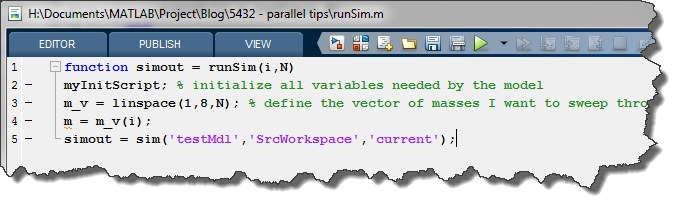# Tips for simulating models in parallel

Update: In MATLAB R2017a the function PARSIM got introduced. For a better experience simulating models in parallel, we recommend using PARSIM instead of SIM inside parfor. See the more recent blog post Simulating models in parallel made easy with parsim for more details.

---------------

As I mentioned many times on this blog, running simulation in parallel using the Parallel Computing Toolbox can save you a lot of time. Today I want to share a few tips to help you getting started with running simulations in parallel.

Serial Parameter Sweep

Before going parallel, let's first look at the standard loop simulating a model. There are tons of ways to setup MATLAB and Simulink to do a parameter sweep. The most common simple setup I see is the following, where we do:

• Initialize the MATLAB base workspace using a script
• Define a vector of values over which you want to sweep
• Inside the loop, index into the parameter vector
• Simulate using sim

For this example, let's use a simple mass-spring-damper model simulation.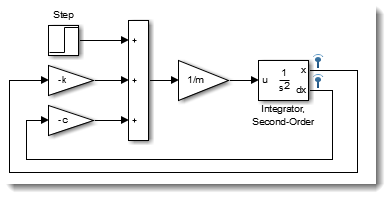We have an initialization script that initializes three variables.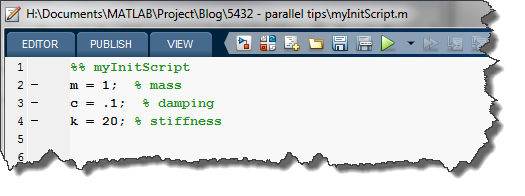And we do the parameter sweep using this code.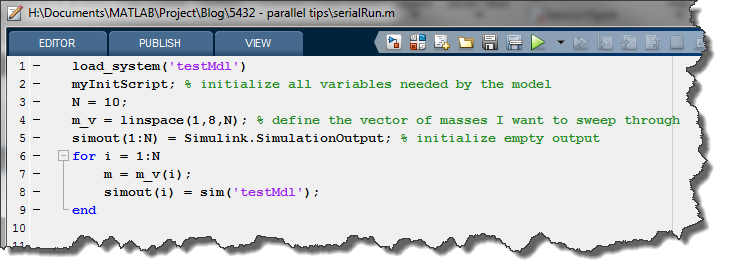When simulating models inside parfor, there are a few intricacies about how parfor manages variables that need to be taken into account. To begin on that topic, I recommend going through the documentation pages about Classification of Variables in parfor-Loops, and Transparency.

Let me resume in a few bullets the important lessons you will learn in those documentation pages:

• Parallel workers are independent MATLAB sessions to which parfor sends code and data to process.
• For efficiency, parfor classifies variables in many categories and sends only the ones it sees as needed
• The code sent to the workers is not executed in their base workspace, but in a special function workspace
• Variables needed by a Simulink model are not "visible" to parfor, and consequently not sent automatically to the workers
• By default, Simulink looks in the base workspace for the variables it needs

Because of those facts, making simulink run inside parfor requires a few tricks. Let's see two techniques to simulate model inside parfor.

Parameter Sweep in the Base Workspace

In this first technique, we counteract the fact that Simulink is not transparent by violating transparency using evalin and/or assignin. Yes... I like to live dangerously!

To be as efficient as possible, we first use an spmd statement to put in the worker's base workspace the data needed by the model that remains constant during the parameter sweep. Then inside the parfor loop, we put in the base workspace the data that changes every iteration, and call sim.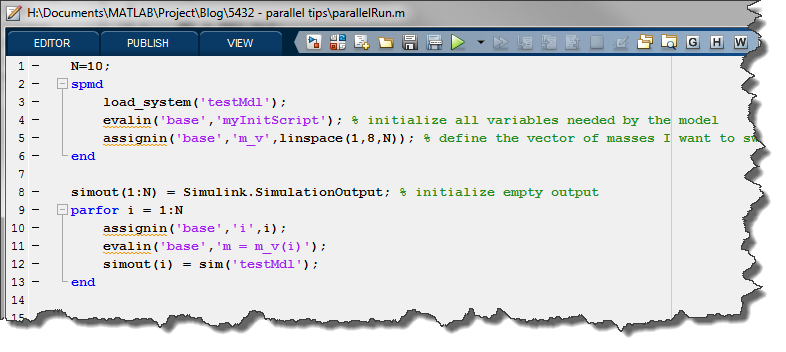Parameter Sweep in a Function Workspace

In this second technique, we do the opposite. We hide the fact that Simulink is not transparent by doing everything inside a function workspace.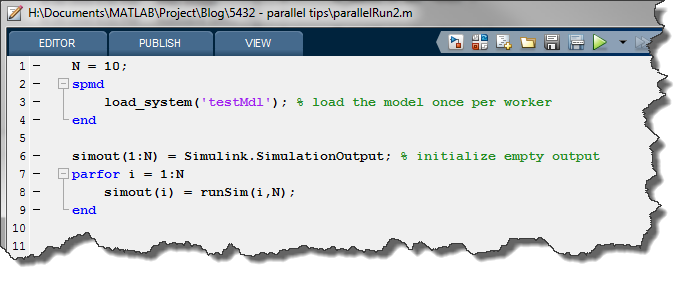Where the function calling sim sets the SrcWorkspace parameter to point to the current workspace: